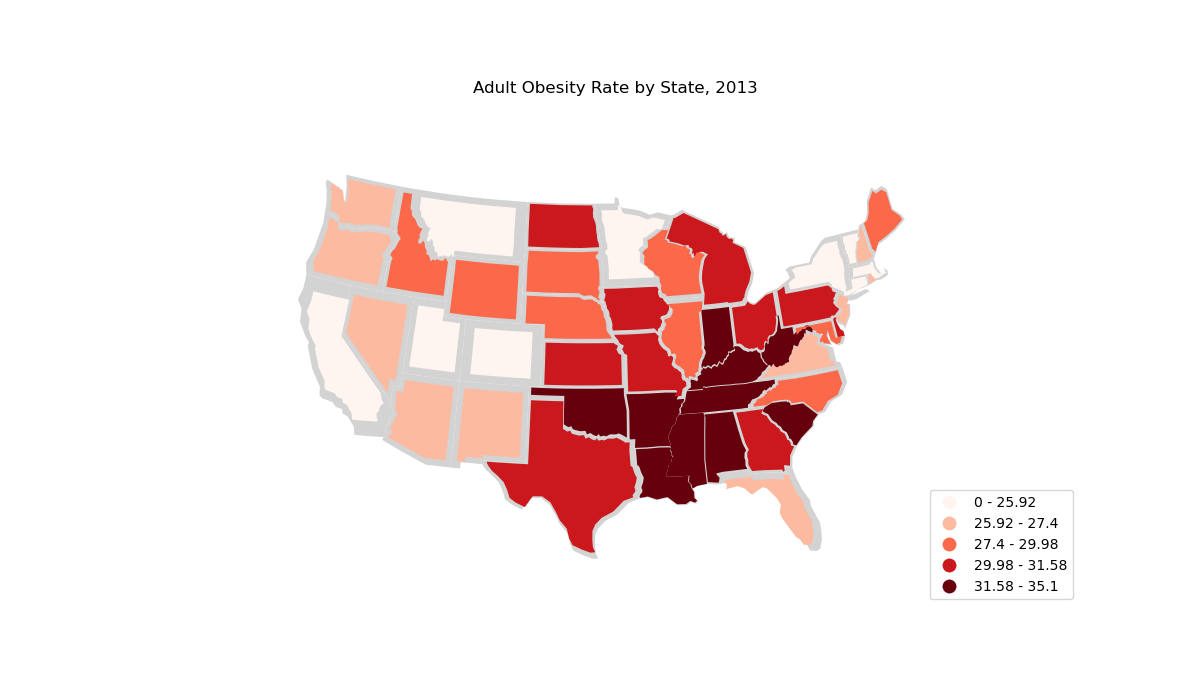# Cartogram of US states by obesity rate¶

This example `cartogram` showcases regional trends for obesity in the United States. Rugged mountain states are the healthiest; the deep South, the unhealthiest.

This example inspired by the “Non-Contiguous Cartogram” example in the D3.JS example gallery.Out:

```Text(0.5, 1.0, 'Adult Obesity Rate by State, 2013')
```

```import pandas as pd
import geopandas as gpd
import geoplot as gplt
import geoplot.crs as gcrs
import matplotlib.pyplot as plt
import mapclassify as mc

contiguous_usa['Obesity Rate'] = contiguous_usa['state'].map(
lambda state: obesity_by_state.query("State == @state").iloc['Percent']
)
scheme = mc.Quantiles(contiguous_usa['Obesity Rate'], k=5)

ax = gplt.cartogram(
contiguous_usa,
scale='Obesity Rate', limits=(0.75, 1),
projection=gcrs.AlbersEqualArea(central_longitude=-98, central_latitude=39.5),
hue='Obesity Rate', cmap='Reds', scheme=scheme,
linewidth=0.5,
legend=True, legend_kwargs={'loc': 'lower right'}, legend_var='hue',
figsize=(12, 7)
)
gplt.polyplot(contiguous_usa, facecolor='lightgray', edgecolor='None', ax=ax)

plt.title("Adult Obesity Rate by State, 2013")
```

Total running time of the script: ( 0 minutes 0.625 seconds)

Gallery generated by Sphinx-Gallery